Clutch Prep is now a part of Pearson
Ch.15 - Acid and Base EquilibriumWorksheetSee all chapters

# Lewis Acid and Base

See all sections
Sections
Identifying Acids and Bases
Arrhenius Acid and Base
Bronsted Lowry Acid and Base
Amphoteric
Lewis Acid and Base
pH and pOH
Ka and Kb
Ionic Salts
Diprotic Acid
Polyprotic Acid

In the 1920s, Gilbert Lewis proposed a new set of definitions for acids and bases.

###### Understanding Lewis Acids and Bases

According to the Lewis definition an acid is an electron pair acceptor.

Concept #1: Understanding Lewis Acids.

Transcript

Hey guys, in this new video, we're going to take a look at the last and final type of acids and bases – the Lewis acid and the Lewis base. So, let's take a look. We’re going to say in the 1920s, we had Gilbert Lewis, he proposed his own new definition of acids and bases. He basically did this almost to the same exact time as Bronsted and Lowry. Here he defined a Lewis acid as something that accepts electrons. So we’re going to say a Lewis acid is an electron acceptor. To understand a Lewis acid, we have to basically figure out what are the different types of Lewis acids. The first one, we say if we have H acts as a Lewis acid, when connected to a very electronegative element. The electronegative elements are oxygen, nitrogen, sulfur, phosphorus or the halogens, group 7A. I'm talking about fluorine, chlorine, bromine or iodine.
Think about it. Let’s think of H being connected to some of these elements. Since all of these elements are more electronegative, they're going to be partially negative. Since H is less electronegative, it's going to be partially positive. We tend to think of this type of Lewis acid when we have H single bonded to the one of these electronegative elements. That's the only time we really look at it and if we're talking about the bond between H and of these electronegative elements. Think about it. Electrons are negatively charged. Since they’re negatively charged, they’ll be attracted to something that's positive or in this case, partially positive. That's what that delta positive means. It means it’s partially positive, a little bit positive. In this case, we're going to say H can act as a Lewis acid.
The second type is anytime we have a positively, fully positively charged hydrogen or metals. Here H+ would be an example of a Lewis acid because it accepts electrons. Electrons are negative. It's positive, opposites attract. If we have positive metals, since they’re all positive, they’ll attract negative electrons. Then the last and final type of Lewis acid is anytime you're central element has less than eight valence electrons around it. Remember, this is the octet rule. Our central element, once they have eight electrons, so they could have a similar configuration as the noble gases. But we're going to say group 2A and 3A elements and transition metals when they're in the center tend to have less than eight valence electrons.
For example, we could have boron. It's in group 3A, so it makes three bonds. Remember, for every bond we make, we're sharing those electrons. Here, boron has six electrons around it. It still has room to accept two more. That's why boron trifluoride can act as a Lewis acid because the central element can accept more electrons. We could also have Be connected to two Cls. Here Be has four electrons around it, so it can accept more electrons, another electron pair to get closer to the octet rule. We could have aluminum bromide here. It has six around it as well. Then our transition metal, common ones, zinc. Here it has four electrons around it as well.
This last type Lewis acid is common with groups to 2A, 3A and the transition metals. When they're in the center, they tend to have less than eight electrons and as a result, they serve as Lewis acids. They're going to accept an electron pair.

When hydrogen is connected to an electronegative element such as P, O, N, S or halogens then it gains a partially positive charge, which makes hydrogen act as a Lewis acid.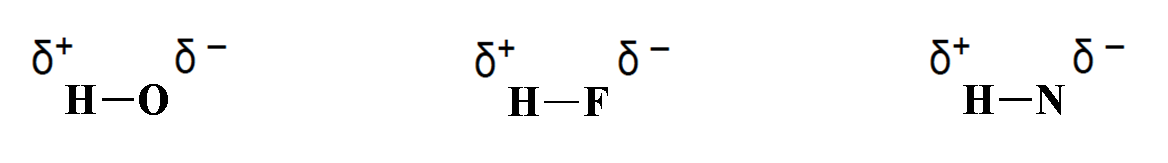Since these metal ions accept negative electron pairs then some Lewis Acids may be positively charged.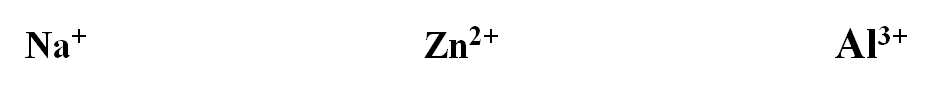If the central element has less than 8 valence electrons around it then it is more likely to accept an electron pair to follow the octet rule.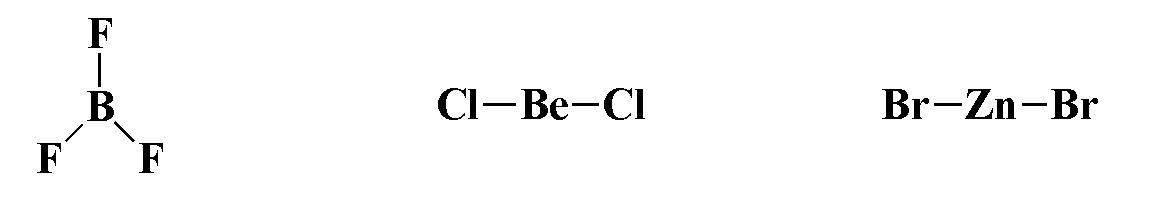According to the Lewis definition a base is an electron pair donor.

Concept #2: Understanding Lewis Bases.

Transcript

If the Lewis acid is an electron pair acceptor, that would have to mean that the Lewis base is an electron pair donor now. What makes Lewis bases? We’re going to say Lewis bases are compounds with lone pairs. See here, all of these, what they have in common is they all have lone pairs. They're all Lewis bases. Then we’re going to say also compounds with, what do all these have in common? They all have in common a negative charge. If you have a negative charge, that means you have excess of extra electrons, so excess of extra lone pairs. Remember, if you're an electron pair donor, you have to have lone pairs and/or a negative charge. Negative charge just means you have extra electrons hanging around. We may not see them but we know they’re there.
This definition is a little bit different than the others and honestly, I think it's the most difficult out of the three because when you get to organic chemistry, organic chemistry is predominantly the chemistry of Lewis acid and bases. That's why this type of theory seems a little bit different from the others because it resides more in the organic chemistry region than general chemistry. But you still need to know it. Remember, a Lewis acid is an electron pair acceptor. A Lewis base is an electron pair donor.

Compounds that have lone pair(s) on a central element can act as Lewis bases.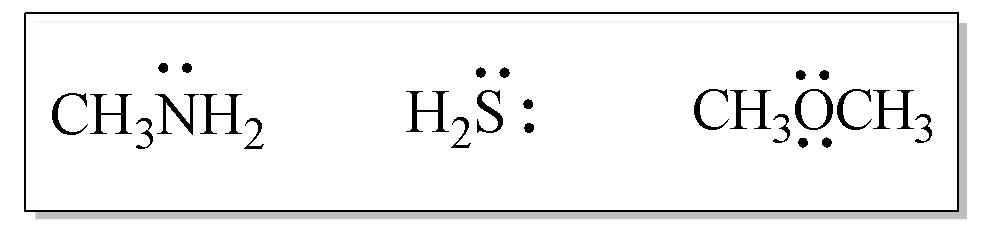Compounds that possess a negative charge can act as Lewis Bases.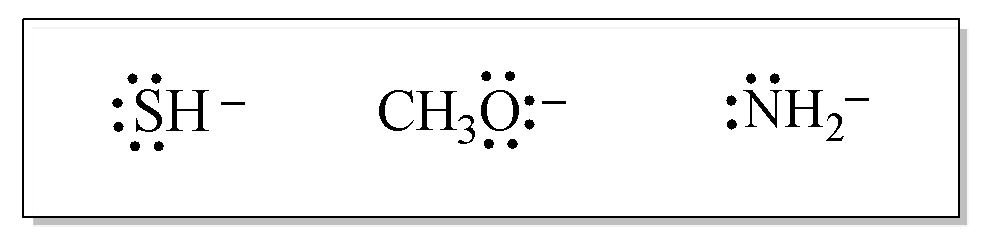Example #1: Identify each of the compounds in the following chemical equation.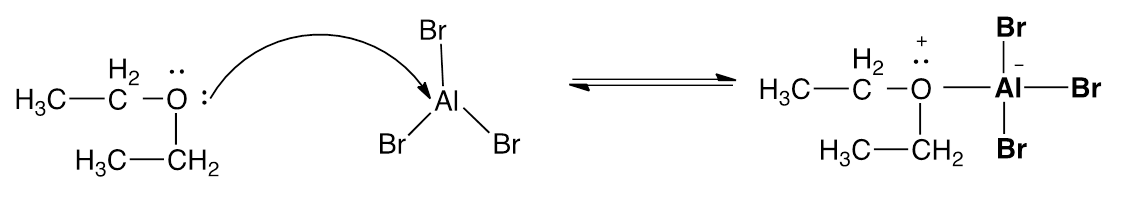Practice: Identify the Lewis acids and bases in the following reactions.

a) H+ + OH ⇌ H2O

b) Cl + BCl3 ⇋ BCl4

c) SO3 + H2O ⇌ H2SO4

Practice: Identify each of the following compounds as either a Lewis acid, a Lewis base or neither.

a)  ZnCl2                                                                                                           b)  CN

c)  NH4+                                                                                                           d) Co3+